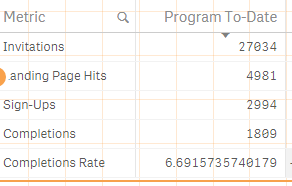# New to Qlik Sense

Discussion board where members can get started with Qlik Sense.

Announcements
Save \$300 - Register for QlikWorld (formerly Qonnections) by January 31st: Learn More
HighlightedNot applicable

## Number formatting

Hi all,

I am using straight table to display some counts.

MetricsDims:

Metrics

Invitations

Landing Page Hits

Sign-Ups

Completions

Completions Rate

];

Using Metrics as dimension.

In dimension field the last one Completions Rate is shown in % all others are counts. so i am not able to format it.

Is their anyway to do it.

You can see in the below image about the issue i am facing.I have selected auto in number formatting, now i need to show only 6.70%  in last row.

Thanks,

Kumar

Tags (1)
1 Solution

Accepted SolutionsMVP & Luminary

## Re: Number formatting

HI,

Try this

=Num(if(Metrics='Invitations',Count({\$<DateType = {invitation},client_name={'Enova'}, CanonicalDate = {">=\$(vEnovaMinDate)<=\$(max(date(CanonicalDate)),-1)"}>}invitation_id),

if(Metrics='Landing Page Hits',count({\$<DateType = {accessed},client_name={'Enova'}, CanonicalDate = {">=\$(vEnovaMinDate)<=\$(max(date(CanonicalDate)),-1)"}>}invitation_id),

if(Metrics='Sign-Ups',sum({\$<DateType = {signed},client_name={'Enova'},CanonicalDate = {">=\$(vEnovaMinDate)<=\$(max(date(CanonicalDate)),-1)"}>}user_signed),

if(Metrics='Completions',sum({\$< DateType = {completion},client_name={'Enova'}, CanonicalDate = {">=\$(vEnovaMinDate)<=\$(max(date(CanonicalDate)),-1)"}>}course_completed),

if(Metrics='Completions Rate',(sum({\$< DateType = {completion},client_name={'Enova'}, CanonicalDate = {">=\$(vEnovaMinDate)<=\$(max(date(CanonicalDate)),-1)"}>}course_completed)/

Count({\$<DateType = {invitation},client_name={'Enova'}, CanonicalDate = {">=\$(vEnovaMinDate)<=\$(max(date(CanonicalDate)),-1)"}>}invitation_id))*100

))))),  if(Metrics='Completions Rate','#,##0.00%', '#,##0'))

Regards

Jagan.

3 RepliesMVP & Luminary

## Re: Number formatting

HI,

Try like this to apply dynamic number formatting

Num(Expression, If(Metric = 'Completions Rate',  '#,##0.00%', '#,##0'))

Hope this helps you.

Regards,

Jagan.Not applicable

## Re: Number formatting

Please check this and correct below expression

Num(Expression,if(Metrics='Invitations',Count({\$<DateType = {invitation},client_name={'Enova'},

CanonicalDate = {">=\$(vEnovaMinDate)<=\$(max(date(CanonicalDate)),-1)"}>}invitation_id),

if(Metrics='Landing Page Hits',count({\$<DateType = {accessed},client_name={'Enova'},

CanonicalDate = {">=\$(vEnovaMinDate)<=\$(max(date(CanonicalDate)),-1)"}>}invitation_id),

if(Metrics='Sign-Ups',sum({\$<DateType = {signed},client_name={'Enova'},

CanonicalDate = {">=\$(vEnovaMinDate)<=\$(max(date(CanonicalDate)),-1)"}>}user_signed),

if(Metrics='Completions',sum({\$< DateType = {completion},client_name={'Enova'},

CanonicalDate = {">=\$(vEnovaMinDate)<=\$(max(date(CanonicalDate)),-1)"}>}course_completed),

if(Metrics='Completions Rate',(sum({\$< DateType = {completion},client_name={'Enova'},

CanonicalDate = {">=\$(vEnovaMinDate)<=\$(max(date(CanonicalDate)),-1)"}>}course_completed)/

Count({\$<DateType = {invitation},client_name={'Enova'},

CanonicalDate = {">=\$(vEnovaMinDate)<=\$(max(date(CanonicalDate)),-1)"}>}invitation_id))*100

)'#,##0.00%'

)))))MVP & Luminary

## Re: Number formatting

HI,

Try this

=Num(if(Metrics='Invitations',Count({\$<DateType = {invitation},client_name={'Enova'}, CanonicalDate = {">=\$(vEnovaMinDate)<=\$(max(date(CanonicalDate)),-1)"}>}invitation_id),

if(Metrics='Landing Page Hits',count({\$<DateType = {accessed},client_name={'Enova'}, CanonicalDate = {">=\$(vEnovaMinDate)<=\$(max(date(CanonicalDate)),-1)"}>}invitation_id),

if(Metrics='Sign-Ups',sum({\$<DateType = {signed},client_name={'Enova'},CanonicalDate = {">=\$(vEnovaMinDate)<=\$(max(date(CanonicalDate)),-1)"}>}user_signed),

if(Metrics='Completions',sum({\$< DateType = {completion},client_name={'Enova'}, CanonicalDate = {">=\$(vEnovaMinDate)<=\$(max(date(CanonicalDate)),-1)"}>}course_completed),

if(Metrics='Completions Rate',(sum({\$< DateType = {completion},client_name={'Enova'}, CanonicalDate = {">=\$(vEnovaMinDate)<=\$(max(date(CanonicalDate)),-1)"}>}course_completed)/

Count({\$<DateType = {invitation},client_name={'Enova'}, CanonicalDate = {">=\$(vEnovaMinDate)<=\$(max(date(CanonicalDate)),-1)"}>}invitation_id))*100

))))),  if(Metrics='Completions Rate','#,##0.00%', '#,##0'))

Regards

Jagan.